# Multiply Decimal Numbers by Whole Numbers (2)

In this worksheet, students multiply one-digit numbers with up to two decimal places by whole numbers.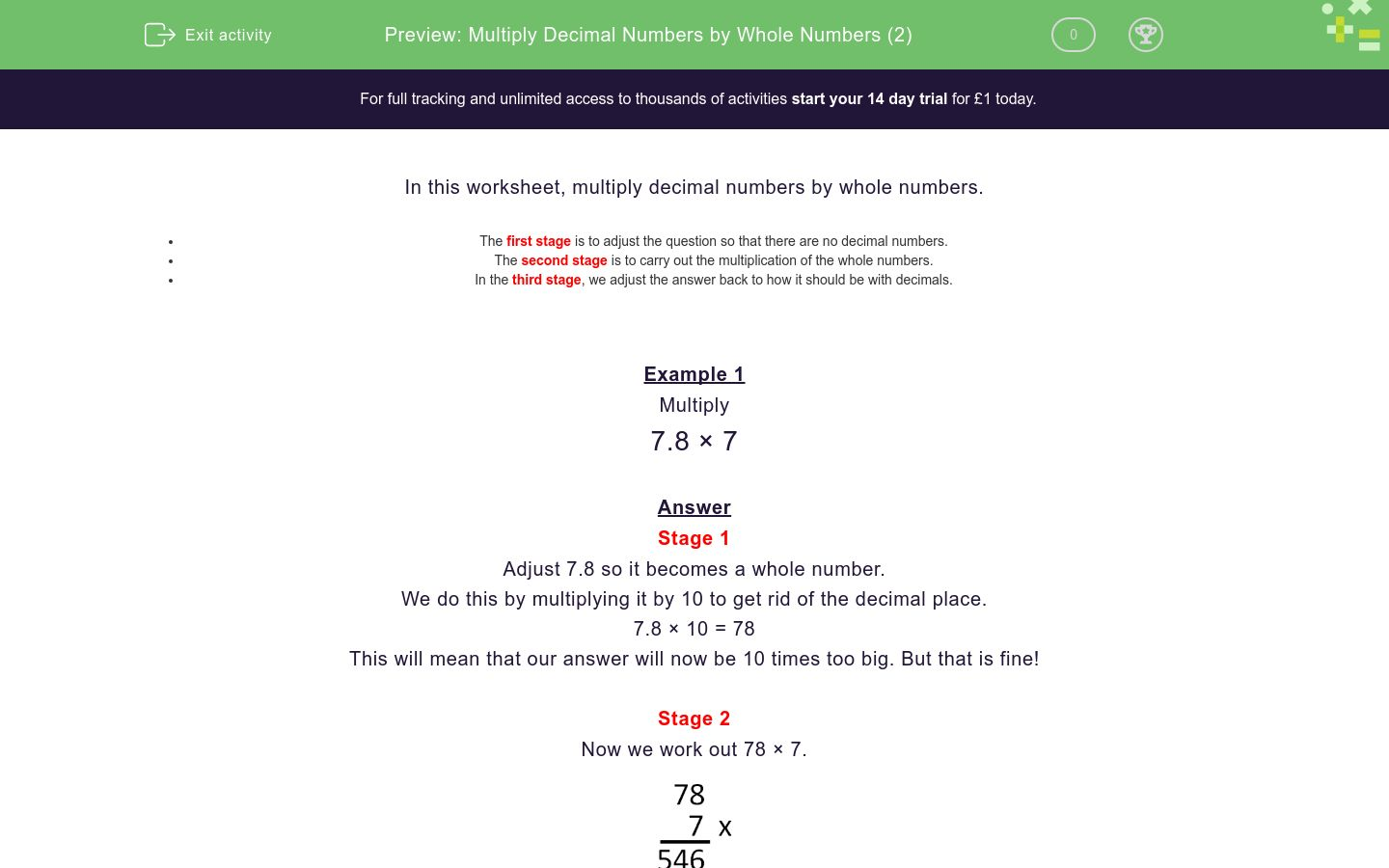Key stage:  KS 2

Curriculum topic:   Number: Fractions, Decimals and Percentages

Curriculum subtopic:   Multiply with Two Decimal Places

Difficulty level:### QUESTION 1 of 10

In this worksheet, multiply decimal numbers by whole numbers.

• The first stage is to adjust the question so that there are no decimal numbers.
• The second stage is to carry out the multiplication of the whole numbers.
• In the third stage, we adjust the answer back to how it should be with decimals.

Example 1

Multiply

7.8 × 7

Stage 1

Adjust 7.8 so it becomes a whole number.

We do this by multiplying it by 10 to get rid of the decimal place.

7.8 × 10 = 78

This will mean that our answer will now be 10 times too big. But that is fine!

Stage 2

Now we work out 78 × 7.Stage 3

Now, remember that this is 10 times too big.

So we divide 546 ÷ 10 = 54.6.

7.8 × 7 = 54.6

Example 2

Multiply

3.68 × 5

Stage 1

Adjust 3.68 so it becomes a whole number.

We do this by multiplying it by 100 to get rid of the 2 decimal places.

3.68 × 100 = 368

This will mean that our answer will now be 100 times too big. But that is fine!

Stage 2

Now we work out 368 × 5.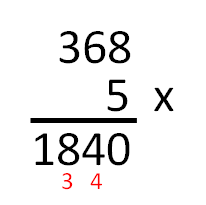Stage 3

Now, remember that this is 100 times too big.

So we divide 1840 ÷ 100 = 18.40 = 18.4.

3.68 × 5 = 18.4

Multiply

4.6 × 6

Multiply

3.6 × 7

Multiply

6.7 × 8

Multiply

4.9 × 7

Multiply

6.8 × 9

Multiply

6.08 × 7

Multiply

7.19 × 8

Multiply

5.93 × 8

Multiply

8.88 × 9

Multiply

9.47 × 7

• Question 1

Multiply

4.6 × 6

27.6
EDDIE SAYS
Multiply 4.6 by 10 to get• Question 2

Multiply

3.6 × 7

25.2
EDDIE SAYS
Multiply 3.6 by 10 to get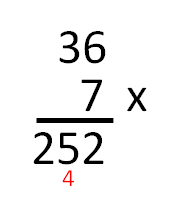• Question 3

Multiply

6.7 × 8

53.6
EDDIE SAYS
Multiply 6.7 by 10 to get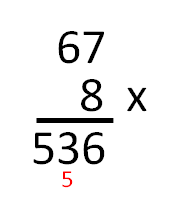• Question 4

Multiply

4.9 × 7

34.3
EDDIE SAYS
Multiply 4.9 by 10 to get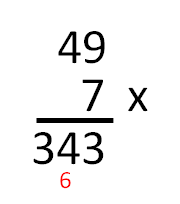• Question 5

Multiply

6.8 × 9

61.2
EDDIE SAYS
Multiply 6.8 by 10 to get• Question 6

Multiply

6.08 × 7

42.56
EDDIE SAYS
Multiply 6.08 by 100 to get• Question 7

Multiply

7.19 × 8

57.52
EDDIE SAYS
Multiply 7.19 by 100 to get• Question 8

Multiply

5.93 × 8

47.44
EDDIE SAYS
Multiply 5.93 by 100 to get• Question 9

Multiply

8.88 × 9

79.92
EDDIE SAYS
Multiply 8.88 by 100 to get• Question 10

Multiply

9.47 × 7

66.29
EDDIE SAYS
Multiply 9.47 by 100 to get